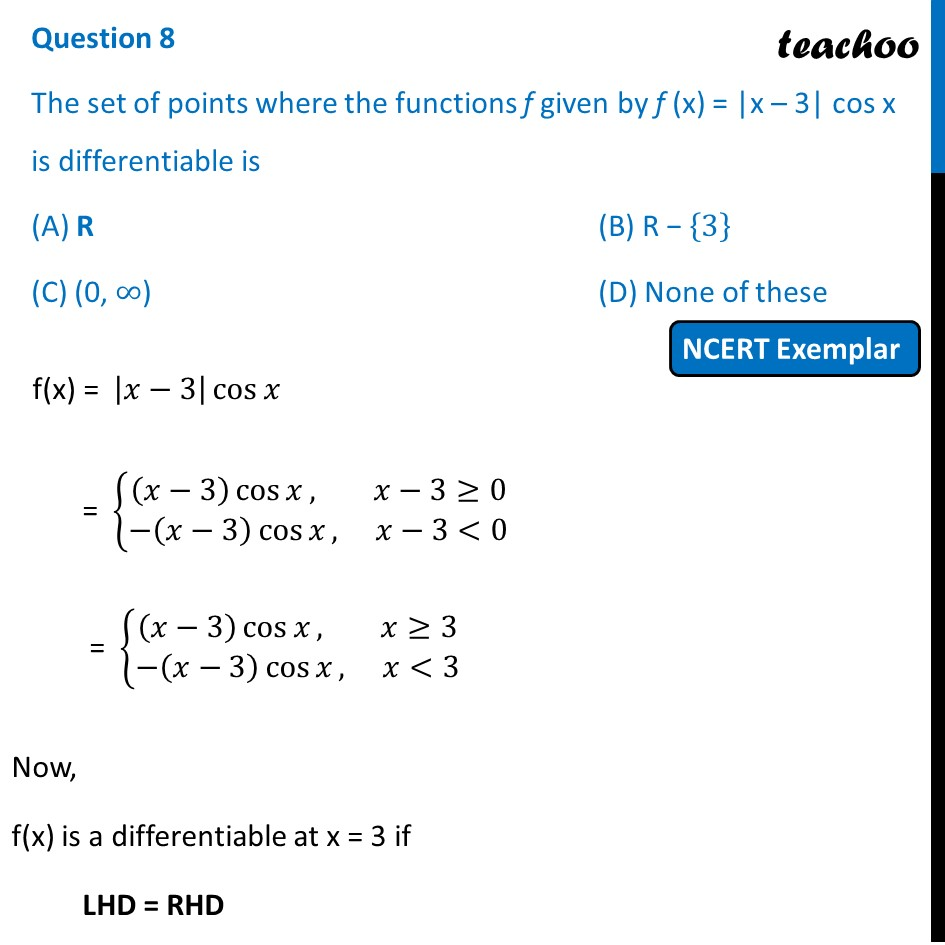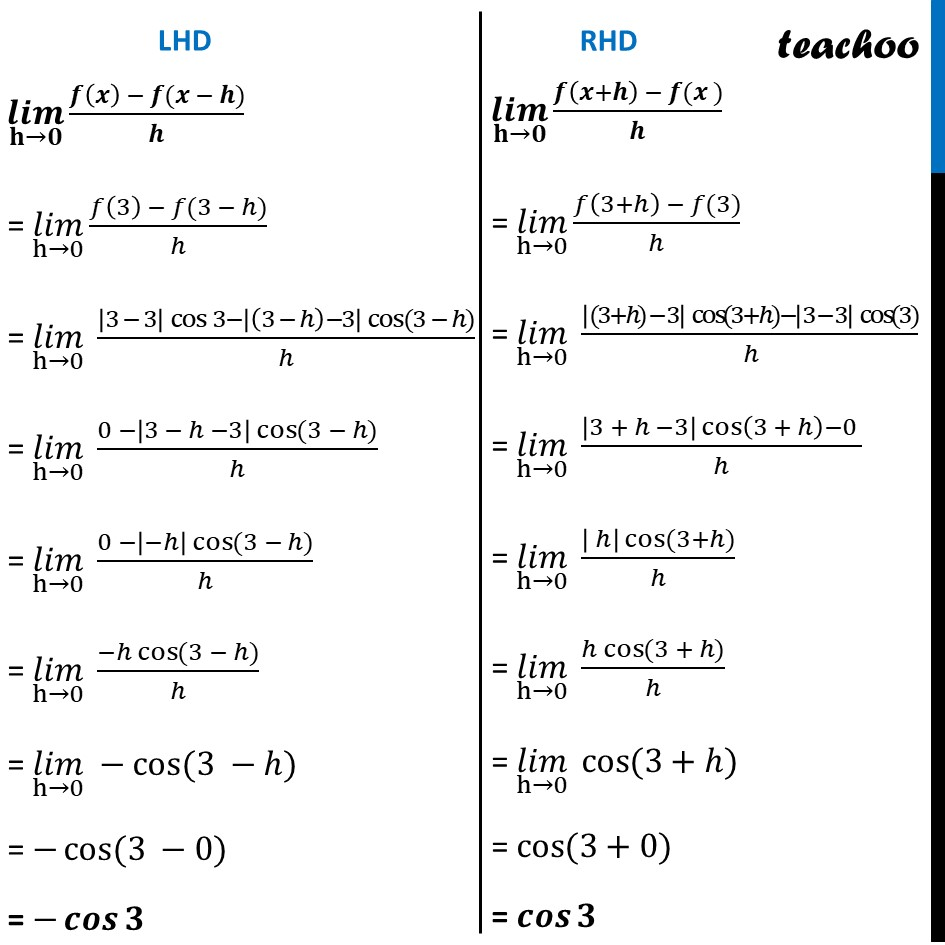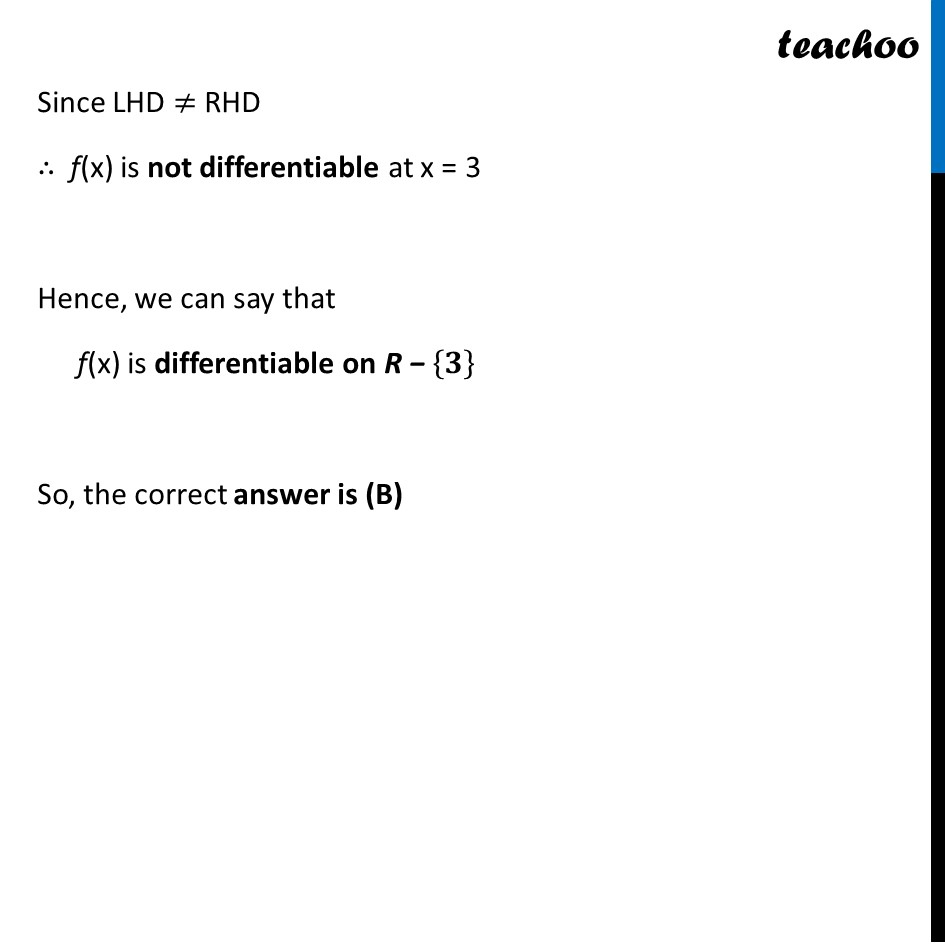NCERT Exemplar - MCQs

Chapter 5 Class 12 Continuity and Differentiability
Serial order wise

## (D) None of these

This question is similar to Ex 5.2, 9 - Chapter 5 Class 12 - Continuity and DifferentiabilityGet live Maths 1-on-1 Classs - Class 6 to 12

### Transcript

Question 8 The set of points where the functions f given by f (x) = |x – 3| cos x is differentiable is (A) R (B) R − {3} (C) (0, ∞) (D) None of these f(x) = |𝑥−3| cos⁡𝑥 = {█((𝑥−3) cos⁡𝑥, 𝑥−3≥[email protected]−(𝑥−3) cos⁡𝑥, 𝑥−3<0)┤ = {█((𝑥−3) cos⁡𝑥, 𝑥≥[email protected]−(𝑥−3) cos⁡𝑥, 𝑥<3)┤ Now, f(x) is a differentiable at x = 3 if LHD = RHD (𝒍𝒊𝒎)┬(𝐡→𝟎) (𝒇(𝒙) − 𝒇(𝒙 − 𝒉))/𝒉 = (𝑙𝑖𝑚)┬(h→0) (𝑓(3) − 𝑓(3 − ℎ))/ℎ = (𝑙𝑖𝑚)┬(h→0) (|3 − 3| cos⁡3−|(3 − ℎ)−3| cos⁡〖(3 − ℎ)〗)/ℎ = (𝑙𝑖𝑚)┬(h→0) (0 −|3 − ℎ −3| cos⁡〖(3 − ℎ)〗)/ℎ = (𝑙𝑖𝑚)┬(h→0) (0 −|−ℎ| cos⁡〖(3 − ℎ)〗)/ℎ = (𝑙𝑖𝑚)┬(h→0) (−ℎ cos⁡〖(3 − ℎ)〗)/ℎ = (𝑙𝑖𝑚)┬(h→0) −cos⁡〖(3 −ℎ)〗 = −cos⁡〖(3 −0)〗 = −𝒄𝒐𝒔⁡𝟑 (𝒍𝒊𝒎)┬(𝐡→𝟎) (𝒇(𝒙+𝒉) − 𝒇(𝒙 ))/𝒉 = (𝑙𝑖𝑚)┬(h→0) (𝑓(3+ℎ) − 𝑓(3))/ℎ = (𝑙𝑖𝑚)┬(h→0) (|(3+ℎ) − 3| cos⁡〖(3+ℎ)〗−|3 − 3| cos⁡〖(3)〗)/ℎ = (𝑙𝑖𝑚)┬(h→0) (|3 + ℎ −3| cos⁡(3 + ℎ)−0 )/ℎ = (𝑙𝑖𝑚)┬(h→0) (| ℎ| cos⁡〖(3+ℎ)〗)/ℎ = (𝑙𝑖𝑚)┬(h→0) (ℎ cos⁡〖(3 + ℎ)〗)/ℎ = (𝑙𝑖𝑚)┬(h→0) cos⁡〖(3+ℎ)〗 = cos⁡〖(3+0)〗 = 𝒄𝒐𝒔⁡𝟑 Since LHD ≠ RHD ∴ f(x) is not differentiable at x = 3 Hence, we can say that f(x) is differentiable on R − {𝟑} So, the correct answer is (B)